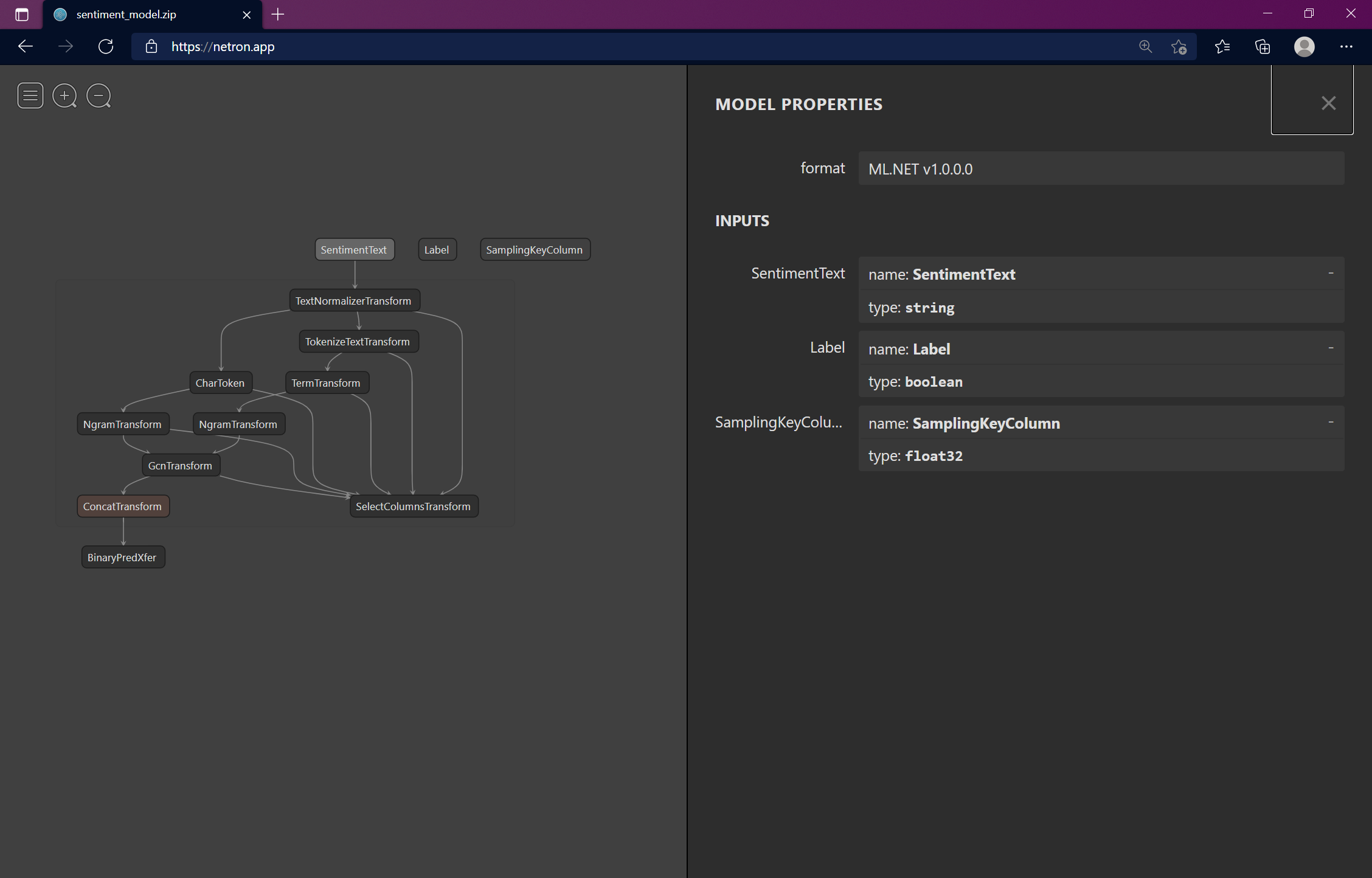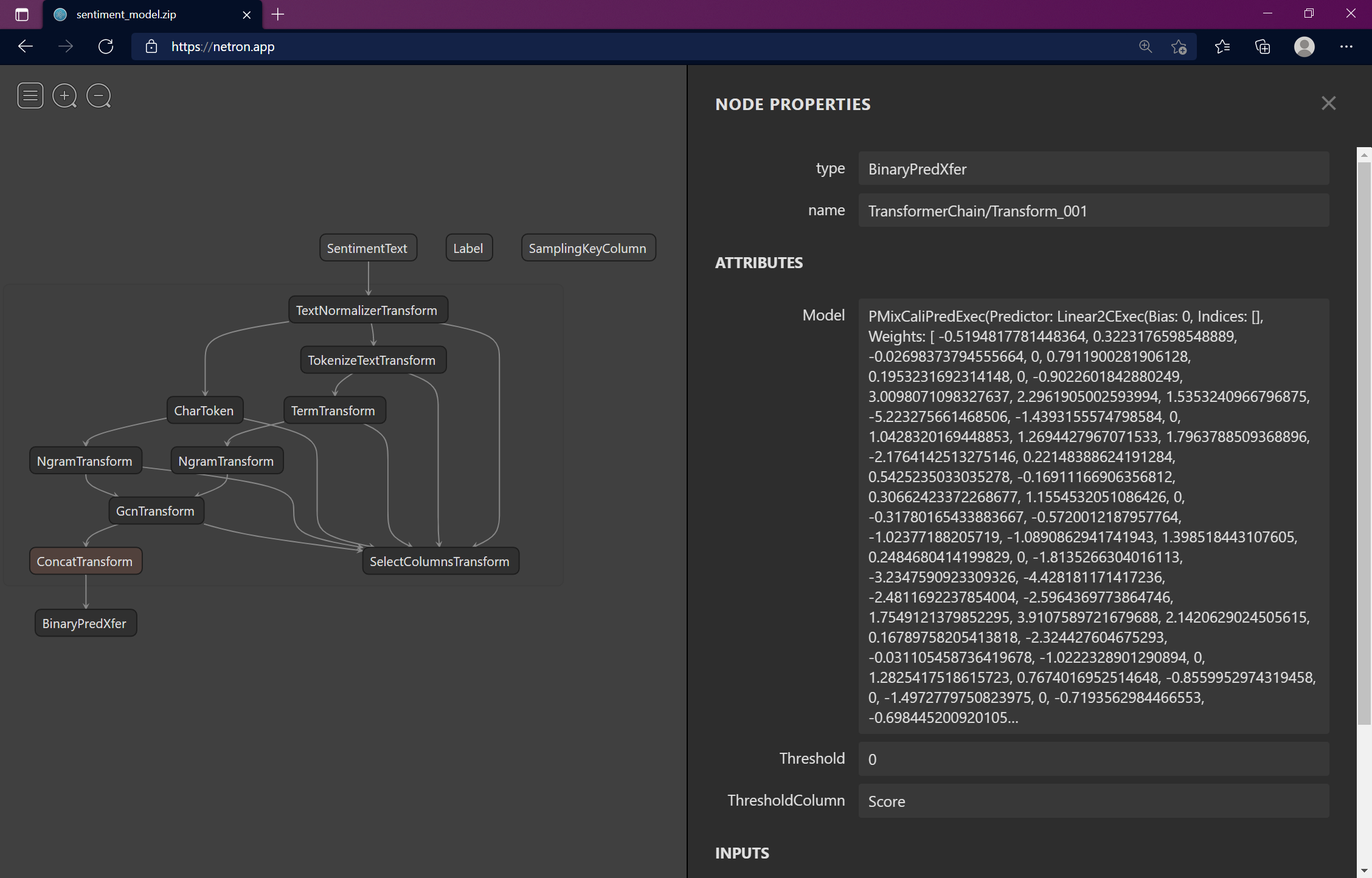## Introduction

Once you've trained a machine learning model, you typically serialize it and save it to a file. This serialized file contains information such as the model inputs and output schema (names, data types), the transformations and algorithms used by the model, weights / coefficients, hyperparameters, and all other sorts of information about the model. The model file is then embedded in an end-user application such as a web API which deserializes the contents of the file and uses the model to make predictions on new data.

How the model is serialized depends on the framework you use to train your model. To standardize model serialization and interoperability, you can use something like Open Neural Network Exchange (ONNX) to represent your models if supported by the framework you're using. That's beyond the scope of this post though.

Regardless of which framework or serialization format is used, if you were to open up the model file, the contents of the model file are often not human readable or difficult to interpret. When the person who trains the model is also putting it into production, they know the format input data needs to be in to make predictions. That's not often the case though. As a result, the people putting models into production need the ability to inspect a model to get a sense of how they need to collect and preprocess input data before making predictions. That's where Netron comes in. Netron is a "visualizer for neural network, deep learning, and machine learning models". In this post, I'll show how you can use Netron to inspect ML.NET models and use that information to define the model input and output schemas.

## Inspecting an ML.NET model

ML.NET models are typically serialized and saved to files with the .zip file extension. Using the .zip file extension is standard convention. However, the extension can be whatever makes the most sense to you.

A common question is, what's in the zip file? The easy but vague answer to that question is, a serialized version of the model. Using Netron, you can go deeper and see exactly what is inside the zip file.

In this post, I'm using a pretrained ML.NET model that classifies sentiment. The model can be found in the dotnet/samples repo. The same concept applies to any other ML.NET model.

To inspect the ML.NET model using Netron:

2. Navigate to https://netron.app. Alternatively, if you'd prefer to use Netron offline, you can also download the latest version of Netron for your operating system (Windows, Mac, or Linux). In this post, I use the web app.

3. Select Open Model... and use the file browser to select your ML.NET model. In this case, our model is sentiment_model.zip.

4. After a few seconds, a graph describing you model appears. How long it takes for your model depends on its size. The larger your model, the longer it takes to load. The nodes in the graph represent the model inputs, transformations, algorithm, and outputs.

5. Usually the top nodes represent the model inputs and the last node represents the algorithm or trainer. Click on any of the top nodes to display more information about the inputs.For this model, we see that there are 3 input properties or columns:

• SentimentText (string)
• Label (boolean)
• SamplingKeyColumn (float32)

Using this information, we can represent the model inputs as a Plain-Old-CLR-Object (POCO) in our end-user application.

``````public class ModelInput
{
public string SentimentText {get;set;}
public bool Label {get;set;}
public float SamplingKeyColumn {get;set;}
}
``````Looking at the last node `BinaryPredXfer`, we see that the algorithm used is for binary classification or predictions. Looking at the ML.NET tasks documentation, we expect to get at least two columns in the prediction output:

• Score (Single)
• PredictedLabel (boolean)

Like the input, we can also represent model outputs or predictions as follows:

``````public class ModelOutput
{
public float Score {get;set;}
public bool PredictedLabel {get;set;}
}
``````

Keep in mind that the name of the class can be anything so long as the properties or column names and types match with those expected by the model.